# Shaft bending, learning the l3/d4 formula

SHAFT BENDING, LEARNING THE L3/D4 FORMULA S039

When a centrifugal volute type pump is operating at its best efficiency point (BEP) the bending forces are evenly distributed around the impeller.

If the pump discharge is throttled from this best efficiency point (BEP) then the fluid velocity recirculating through the cutwater will increase, causing a reduction in pressure, and you will experience an increase in force at approximately 240 degrees from the cutwater in the direction of shaft rotation.

It also follows that if the pump capacity increases because of a lack of sufficient head then this increase in flow will cause a decrease in pressure and an increase in force in the opposite direction, at approximately 60 degrees from the cutwater.• The illustration shows the direction of these forces.
• Please note that the degrees are measured from the pump cutwater (The lip that directs the flow out the discharge) in the direction of shaft rotation

The amount of force that is generated can be calculated from the following formula:

THE BENDING FORCE FORMULA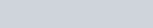• P = Pounds of force being generated
• K = Radial thrust factor 0.3 to 0.35 (see chart A1 below)
• H = Total head @ B.E.P.
• Sg. = Specific Gravity of the liquid
• B2 = Width of the impeller including the shrouds (walls)
• D2 = Outside diameter of the impeller (inches)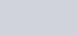• Q = Gallons per minute actually pumping
• Qn = Gpm @ the B.E.P.

METRIC BENDING FORCE FORMULA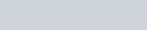• P= Kilograms of force being generated
• K= Radial thrust factor 0.3 to 0.35 (See chart A1 below)
• Sg. = Specific Gravity of the liquid
• H = Total head @ best efficiency point (BEP) (meters)
• B2 = Width of the impeller including shrouds (walls) (cm)
• D2 = O.D. of the impeller (cm)• Q= M3/hr. actually pumping
• Qn= M3/hr. @ the best efficiency point (BEP).

• The radial load is the greatest at shut off when Q = “0” and Kq = 1 (see the above drawing). Note that the thrust is at 240 degrees from the cutwater, in the direction of shaft rotation.
• As capacity Q increases, Kq and the radial load decreases to “0” at the best efficiency point where Q = Qn.
• As capacity Q increases to a value greater than Qn, Kq and the resultant load increases as negative values. The load is now in the opposite direction, or 60 degreesIn the above graph the term specific speed describes the shape of the impeller.

CALCULATING SHAFT DEFLECTIONThe formula for the calculation of a multi-diameter shaft looks like this: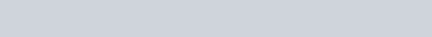• Y = Shaft deflection at the impeller center line measured in inches
• F = Hydraulic Radial imbalance, pounds ( “P” in the previous calculation)
• M & N = Distances from the impeller centerline to the steps on the shaft, inches
• L = Distance from impeller centerline to centerline of the inboard bearing, inches
• X = Span between bearing centerlines, inches
• IL, IM, IN, IX = Moments of inertia of the various diameters, inches
• E = Modulus of elasticity of the shaft material (psi.)

How much the shaft bends depends upon the length of the shaft and its diameter. The strength of the shaft has nothing to do with this. The strength only determines if the shaft will break.

The following formula is a simplified version of this longer formula. It is the formula we use for a single stage centrifugal pump with a solid, round shaft and an over hung impeller.

You will note that shaft strength does not enter into the formula. The important number is the Modulus Of Elasticity (E), and as you will learn just about every shaft we use in the pumping business has the same modulus.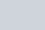• F = The force we calculated in the previous formula plus the weight of the impeller.
• L = The length of the shaft from the center of the inboard bearing to the center of the impeller.
• 3 = A factor used for an end suction centrifugal pump. A double-ended design would use a different number
• E = Modulus of elasticity; 28 to 30 x 106 psi. ( 0,196 to 0,201 X 106 N/mm2) for most metals with the exception of Titanium
• I = Moment of inertia for a solid, round shaft =
•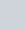Substuting the Moment of Inertia in to the formula gives us: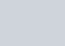Since the F is the same in both pumps, along with 3, Π and 64; and since the Modulus of Elasticity is just about the same for all shaft materials, we can cancel out those terms and we are left with Y = L/D4 This ratio then becomes a logical method of comparing two competing pumps that have different shaft sizes. This ratio is often called the stiffness ratio, slenderness ratio, or the flexibility factor.

If we assume that the length of the shaft from the center of the inboard bearing to the center of the impeller is a fixed amount (8 inches or 200 mm.) then we can easily see the affect of varying the shaft diameter in the stuffing box area. The following table shows the relationship:

STIFFNESS RATIO

L = Assume 8″ (200 mm) SHAFT

 Diameter L3/D4 Diameter L3/D4 1″ 512 25 mm 20.48 1 3/8″ 143 35 mm 5.33 1 1/2″ 101 38 mm 3.84 1 3/4″ 55 45 mm 1.95 1 7/8″ 42 48 mm 1.51 2″ 32 50 mm 50

If you keep this ratio below 60 (2 in the metric system) you will not have too much trouble with shaft bending. If, however, you do not have a low L3/D4 you will have problems with the shaft packing, mechanical seals and the pump bearings. Keep in mind that we are measuring the shaft diameter. If there is a sleeve on the shaft, do not measure the diameter of the sleeve.

Pump packing has a very poor memory and is not able to follow the bending or deflection of a badly designed shaft. Some packings can be readjusted for the changing leak rate, but in almost every case additional heat will be generated requiring even more flush water to remove the extra heat. If the packing is not re-adjusted with the changing of the pump discharge head excessive leakage will follow and along with it, all of the problems associated with too much leakage.

A bending shaft can be deadly to a mechanical seal because it increases the opportunity for the rotating part of the seal to contact a stationary portion of the pump causing the lapped seal faces to open and let solids penetrate. If we can keep the lapped seal faces together the seal will not leak and solids cannot penetrate between them. The more shaft movement we have the more likely the faces are to open.

Bearings are affected by the shaft movement in the same way they are affected by pump to motor misalignment. There will be an increase in the bearing loading and a corresponding increase in the lubricating oil temperature.

Another problem with shaft movement is often overlooked. The pump has several critical tolerances and shaft movement changes them. The most obvious are wear ring clearance, impeller clearance, bearing fit, and seal face loading. These changes can cause additional heat generation, loss of capacity and loss of efficiency.

In summary then, shaft deflection is certainly undesirable. If the L3/D4 is too high you will not be able to operate in slight cavitation or very far from the BEP. (best efficiency point) and in the real world that is not very practical.

Be especially careful with most small pumps, they frequently operate above 3000 rpm and their L3/D4 ratio is atrocious.

### Posted

• On February 17, 2018